## Suppose the radius of a circle is three units what is the circumference

Question

Suppose the radius of a circle is three units what is the circumference

in progress 0
2 months 2021-08-02T19:12:11+00:00 1 Answers 2 views 0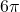or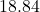units
The circumference of a circle can be found using the formula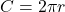, where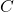is the circle’s circumference and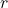is the circle’s radius. We know that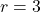and that we are solving for. Therefore: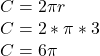Since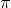is approximately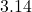,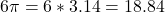units. Hope this helps!